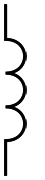# Air Cored Inductor Calculator

This calculator is useful when making antenna matching units, low pass filters, crystal sets, antenna traps, resonant circuits or anywhere where an air cored single layer inductor is required.

Diameter and length of inductor are entered in millimetres along with the number of turns. Frequency can be entered to find the Q of the inductor at a specific frequency, but is not required. Inductance values are calculated to 3 decimal places and are given in nH, µH, mH and even to 5 decimal places, H! An example of a T-match inductor for 1.8MHz is already inputted, click calculate, or clear fields to enter your own values.

 Input coil diameter, length & number of turnsCoil Diameter mm Coil length mm Number of turns t Frequency(Not required, but used for calculating Q) MHz

 Calculated inductance, Q and wire length Nano H nH Micro H µH Milli H mH Henry H Inductor Q Q Wire Length m cm mm

Wire length is calculated by (diameter x π x turns). π is taken to be 3.14159265358979323846264338327950288419716939937510 which is a little over the top I know! As 39 decimal places is sufficient to estimate the circumference of any circle that fits in the observable universe with precision comparable to the radius of a hydrogen atom, the 50 decimal places I have used should be OK for your inductor. Just remember to cut a bit longer for some tails!

1.LOVE YOUR CALULATOR……NOT SURE WHEN I NEED TO USE IT….I AM A HAM VIRGIN….BUT LEARNING…..
THANKS A BUNCH
LARRY

2.Re: Air Cored Inductor Calculator

Hi, Is the coil diameter measured outside, at the middle or inside of the coil ?

Rgds/ 73

Arto Salo
OH1ID

1.Hi.
I never use thick enough wire to worry, but I would use the inside diameter.
73, John.

3.The coil diameter uses the mean diameter of the copper coil.

A 1mm wire wound on a 14mm bobbin will have a mean diameter a bit over 15mm.
The bit over is the uncoiling that naturally occurs when one releases the tensioning force.

I use >=1.6mm diameter enameled copper wire and prefer to use 2.12mm wire wound onto an AA battery.
My mean diameter is roughly 16.9mm
9¾ Turns gives ~1uH

4.Is it possible to add a field for the copper wire diameter?
And use that to calculate the coil resistance in ohms?

1.Perhaps that would be better as a separate calculator.

5.What is the meaning of Coil Diameter and Coil length?

1.Diameter is how wide the coil is and length is how long the coil is.

6.hi tanx for you helping amateur radio in all of the world

i used of air core calculator.

best regards from Tehran-iran
amirhosein 73

7.A good approximate formula (accurate enough to 1%) is:

L = (n . d)(n . d)/l x 10^{-10) Henrys , where n is number of turns, d is diameter in mm, l is length in cms,

8.I love the idea that you have Pi to 50 digits. But. It is false precision in the wire length because the length depends on the Turns Per Inch as well. The true length of the line is the length of the hypotenuse of the triangle formed from the true circumference and the turn spacing. It is an error larger than the diameter of an electron, but I think I can live with your formula! ;-))

1.Very good Bennett! 🙂

9.I just came across this site. I ran some numbers when designing the shunt for my HiQ antenna and found yous to be more accurate than others. My SWR was good without but I wanted to get rid of some unwanted wind noise on digital modes in the especially in the 20 meter band. I used the center of the coil “(ID+OD)/2” which was 37.6mm x 32mm x 10.5 turns. The wire is #8 (3.2mm) dia. My first calculations were less but when I measured it I got 3.1 µH. Yours calculated 3.146. The other calculators I tried online were estimating from 2.2 to 2.8 µH. The wire was some common copper ground wire I picked up at a local hardware store.

Good job on the calculator.
Robert N9NQ

10.in order to make an foldable antenna for 27.205mhz, can i use an wipe of 70 cm an coil (22turns) of 10mm diameter and 45mm long ? where can i make the connection between turns ?

1.It’s hard to tell what you are trying to do. I’ve used this with the loaded quarter wave with good results.
Cheers!